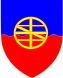FISICAEXE - SOLVED PROBLEMS IN PHYSICSFISICAEXEScientia Scientia

### Presentation

This site aims to present some solved physics exercises. The solutions are presented in the most detailed way possible, this aims not to solve a particular problem but to show a strategy for solutions of any problem of that subject in question. The division in Basic, Average and Advanced levels does not match the official division of education, being only indicative of the difficulty of the exercises. Problems are presented on each page and a link to the solution that opens in a new screen.

#### Basic Level

The exercises in this part aim to show the direct application of basic concepts of physics, are classic exercises using numerical values and direct resolution without the use of more difficult mathematical tools. These problems, in general, are found as examples in physics textbooks. In this part, the physics was divided into four areas, Mechanics, Thermology, Electromagnetism and Optics.

#### Average Level

The exercises solved here are commonly found in books, handouts, college tests, and Physics Olympiads. Many exercises present literal solutions and the mathematical tools used are slightly more elaborate than in the previous level.
Here Physics was divided into seven main branches, Mechanics, Thermodynamics, Electromagnetism, Optics, Oscillations and Waves, Modern Physics and Measurements. The exercises are, in general, of greater difficulty being necessary that the basic knowledge of each subject has already been trained.